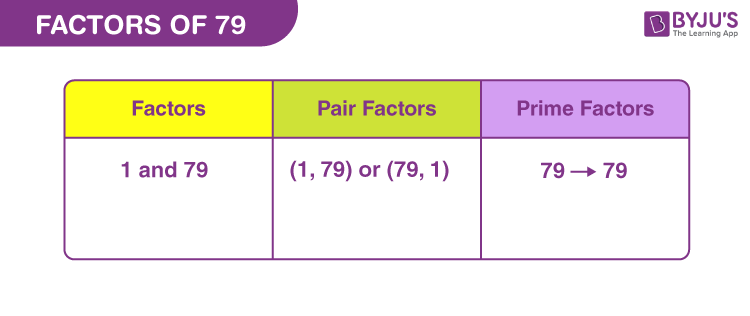# Factors of 79

Factors of 79 divides the original number, evenly or uniformly. It means there is no remainder left after division. These factors are also called divisors of the original number. Since 79 is a prime number, therefore, it will have only two factors.

79 is an odd number but not a perfect square. The multiplication table of 1 and 79 only has the value of 79.## How to Find the Factors of 79?

Factors of a number divide it into equal parts. Thus, the remainder should be zero.

 79 ÷ 1 = 79 [Remainder = 0] 79 ÷ 79 = 1 [Remainder = 0]

Therefore, there are only two factors of 79. They are 1 and 79.

Let us see if 79 is divisible by any other number.

79 ÷ 2 = 39.5

79 ÷ 5 = 15.8

79 ÷ 10 = 7.9

79 ÷ 35 = 2.25714285714

Since, 79 is not divisible by any other number, evenly, therefore apart from 1 and 79, there are no other possible factors.

## Pair Factors of 79

The product of two natural numbers results in 79, to form a pair of factors. Thus, the possible pair factors of 79 are:

• 1 × 79 = 79
• 79 × 1 = 79

Therefore, the pair factors of 79 are (1, 79) or (79, 1).

If we consider pair factors in negative terms, then;

• -1 × -79 = 79
• -79 × -1 = 79

Therefore, the negative pair factors are (-1, -79) or (-79, -1).

## Prime Factorisation of 79

79 is a prime number itself, therefore, prime factorisation of 79 is not possible. 79 is divisible by 79 only.

79 ÷ 79 = 1

 Prime factorisation of 79 = 79 Exponential form = 791

## Solved Examples

Q.1: Find if the sum of factors of 79 is an even number or an odd number.

Solution: The factors of 79 are 1 and 79.

Sum = 1 + 79 = 80

Thus, 80 is an even number.

Q.2: What is the GCF of 67 and 79?

Solution: We have to list the factors of 67 and 79.

67 → 1, 67

79 → 1, 79

Therefore, the GCF of 67 and 79 is 1.

Q.3: What are the common factors of 79 and 237?

Solution: The factors of the respective numbers are:

79 → 1, 79

237 → 1, 3, 79, 237

Therefore, the common factors of 79 and 237 are 1 and 79.

### Practice Questions

1. What is the average of factors of 79?
2. How many factors does the number 79 have?
3. What is the value of 79 multiplied by 50?
4. Do 79 and 71 have the same number of factors?

## Frequently Asked Questions on Factors of 79

### What are the factors of 79?

The factors of 79 are 1 and 79.

### Is 79 divisible by any other number?

79 is divisible by only 1 and 79, but not by any other factor.

### Is 79 a prime number?

Yes, 79 is a prime number because it has only two factors.

### What are the multiples of 79?

The multiples of 79 are 79, 158, 237, 316, 395, 474, 553, 632, 711, 790 and so on.

### What are the factor pairs of 79?

Answer: The factor pair of 79 is (1,79) or (79,1)

1 x 79 = 79